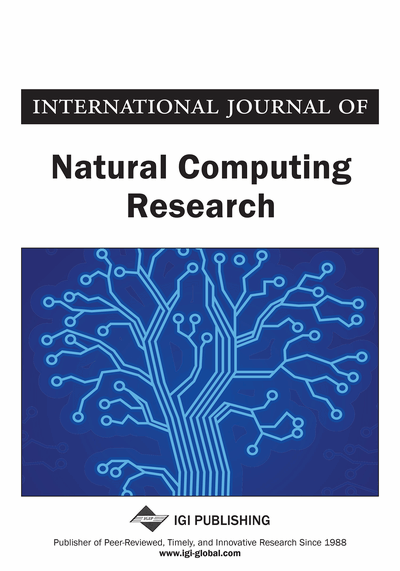A Parallel Hardware Architecture based on Node-Depth Encoding to Solve Network Design Problems

Marcilyanne M. Gois (Escola de Engenharia de São Carlos (EESC), University of São Paulo (USP), São Carlos, São Paulo, Brazil), Paulo Matias (Instituto de Física de São Carlos (IFSC), University of São Paulo (USP), São Carlos, São Paulo, Brazil), André B. Perina (Instituto de Ciências Matemáticas e de Computação (ICMC), University of São Paulo (USP), São Carlos, São Paulo, Brazil), Vanderlei Bonato (Instituto de Ciências Matemáticas e de Computação (ICMC), University of São Paulo (USP), São Carlos, São Paulo, Brazil) and Alexandre C. B. Delbem (Instituto de Ciências Matemáticas e de Computação (ICMC), University of São Paulo (USP), São Carlos, São Paulo, Brazil)
DOI: 10.4018/ijncr.2014010105
Available
\$37.50
No Current Special Offers

Abstract

Many problems involving network design can be found in the real world, such as electric power circuit planning, telecommunications and phylogenetic trees. In general, solutions for these problems are modeled as forests represented by a graph manipulating thousands or millions of input variables, making it hard to obtain the solutions in a reasonable time. To overcome this restriction, Evolutionary Algorithms (EAs) with dynamic data structures (encodings) have been widely investigated to increase the performance of EAs for Network Design Problems (NDPs). In this context, this paper proposes a parallelization of the node-depth encoding (NDE), a data structure especially designed for NDPs. Based on the NDE the authors have developed a parallel algorithm and a hardware architecture implemented on FPGA (Field-Programmable Gate Array), denominated Hardware Parallelized NDE (HP-NDE). The running times obtained in a general purpose processor (GPP) and the HP-NDE are compared. The results show a significant speedup in relation to the GPP solution, solving NDP in a time limited by a constant. Such time upper bound can be satisfied for any size of network until the hardware resources available on the FPGA are depleted. The authors evaluated the HP-NDE on a Stratix IV FPGA with networks containing up to 2048 nodes.
Article Preview
Top

1. Introduction

Network Design Problems (NDPs) are combinatorial problems that must find the most appropriate network to solve applications of the real world. In general, these problems are modeled as graphs and can be found in the fields of engineering and sciences, as for example, the development of networks for telecommunications (Kershenbaum, 1993; Talbi & Meunier, 2006) design of electric circuits (Deo, 2004), vehicle routing (Golden et al., 2008), computer network applications (Deo, 2004), and phylogenetic trees (Mintz & Bakos, 2012). In the real world, NDPs have large numbers of elements (nodes) and interconnections (edges). Moreover, several constraints restrict the types of connections that an algorithm can use to design a network. For example, to solve a simple NDP called Minimum Spanning Tree Problem (MSTP), we can use polynomial time algorithms such as Prim (1957) and Kruskal (1956). However, the problem becomes NP-Hard if a degree constraint is added, which is known as Degree Constrained Minimum Spanning Tree Problem (dc-MSTP) (Martinez-Perez & Zimmermann, 2009; Garey & Johnson, 1979). In this case, it is very hard to find an adequate solution to the NDP in reasonable running time.

Several other extensions of the MSTP are also NP-Hard. For such NDPs, population-based metaheuristics that simultaneously investigate a large set of potential points of the search space have been researched. Several of them, as Evolutionary Algorithms (EAs), use the strategy of generating many network configurations (points in the search space) for the possible solutions of an NDP, testing whether the trees respect the constraints (Omara & Arafa, 2010).

However, such approach does not often provide satisfactory results for large scale problems, since the generation of a feasible network that respects all the constraints is rare for large networks. To develop efficient EAs for complex large-scale NDPs, new dynamic data structures (encodings) that exclusively generate feasible networks have been researched (Caminiti & Petreschi, 2010; Rothlauf, 2006). Those encodings allow a more suitable exploitation of the search space, increasing the quality of solutions of EAs. Among the encodings from the literature, the node-depth encoding (NDE) (Santos et al., 2010) can design networks in onlyaverage running time, whereas other encodings require at least O(n), O(n log(n)) and(Raidl & Julstrom, 2003a; Santos et al., 2010), where n is the number of graph nodes.

The discovery of the global optimum (or an adequate suboptimum) from a non-trivial large-scale NDP requires the construction and evaluation of thousands or millions of potential spanning trees to find an adequate network. As a consequence, the total running time needed to generate and evaluate all the spanning trees explored by a population-based metaheuristic is a bottleneck for the algorithm performance (dos Santos et al., 2008).

The implementation of a NDE directly in hardware can parallelize EAs properly, reducing their computational time. Thus, an EA with NDE implemented in hardware can enhance the performance of large scale NDPs. FPGAs (Field Programmable Gate Array) provide spatial computation allowing an high degree of parallelism and flexibility for developing solutions totally customized for a given application (Bobda, 2007; Middendorf et al., 2002).

Complete Article List

Search this Journal:
Reset
Open Access Articles: Forthcoming
Volume 11: 4 Issues (2022): Forthcoming, Available for Pre-Order
Volume 10: 4 Issues (2021): 2 Released, 2 Forthcoming
Volume 9: 4 Issues (2020)
Volume 8: 4 Issues (2019)
Volume 7: 4 Issues (2018)
Volume 6: 2 Issues (2017)
Volume 5: 4 Issues (2015)
Volume 4: 4 Issues (2014)
Volume 3: 4 Issues (2012)
Volume 2: 4 Issues (2011)
Volume 1: 4 Issues (2010)
View Complete Journal Contents Listing